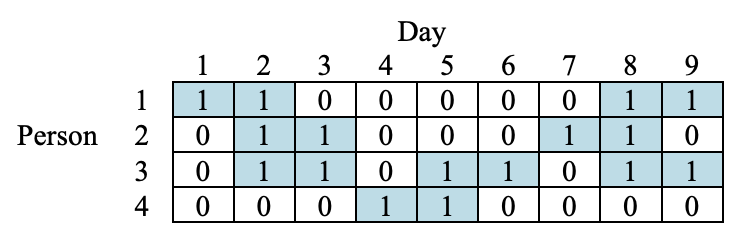시간 제한메모리 제한제출정답맞힌 사람정답 비율
2 초 512 MB51015513633.415%

## 문제

ICPC manager plans a new project which is to be carried out for n days. In this project, m persons numbered from 1 to m are supposed to work. Each day j (1 ≤ jn) requires dj persons, and each person i (1 ≤ im) wants to work wi days.

To increase the efficiency in performing the project, the following two conditions should be satisfied:

1. each person works for only consecutive w days when he/she works, and
2. each person can work again after he/she has a rest for at least h days.

ICPC manager wants to find a working plan to assign the working days for all persons such that the number of working days of each person i (1 ≤ im) is equal to wi and the number of persons who work for each day j (1 ≤ jn) is equal to dj, and above two conditions are also satisfied.

For example, assume the project is carried out for n = 9 days, and m = 4 persons participate in the project. Let w = 2 and h = 1. Also, assume (w1, w2w3w4) = (4, 4, 6, 2) and (d1, d2, d3, d4, d5, d6, d7, d8, d9) = (1, 3, 2, 1, 2, 1, 1, 3, 2). The table below shows a feasible solution where the i-th row corresponds to person i, and the j-th column corresponds to day j. If person i works or has a rest in day j, the value of the table element with row i and column j is 1 or 0, respectively.Given m, n, w, h, wi (1 ≤ im) which is a multiple of w, and dj (1 ≤ jn), write a program to find a feasible solution as a working plan.

## 입력

Your program is to read from standard input. The input starts with a line containing four integers, m, n, w, h (1 ≤ m ≤ 2,000, 1 ≤ n ≤ 2,000, 1 ≤ w, hn). The following line contains m integers where the i-th (1 ≤ im) integer represents wi (1 ≤ win) which is a multiple of w. The next line contains n integers where the j-th (1 ≤ jn) integer represents dj (0 ≤ dj ≤ m).

## 출력

Your program is to write to standard output. If there is a feasible working plan, print 1 in the first line followed by m lines, each i-th (1 ≤ im) line should contain wi/w integers. These integers form an increasing sequence of first days that person i works in the feasible plan. If there is no feasible working plan, print only -1 in the first line. The first sample below corresponds to the example given in the table above.

## 예제 입력 1

4 9 2 1
4 4 6 2
1 3 2 1 2 1 1 3 2


## 예제 출력 1

1
1 8
2 7
2 5 8
4


## 예제 입력 2

4 7 2 2
4 4 4 2
1 3 2 1 3 3 1


## 예제 출력 2

-1